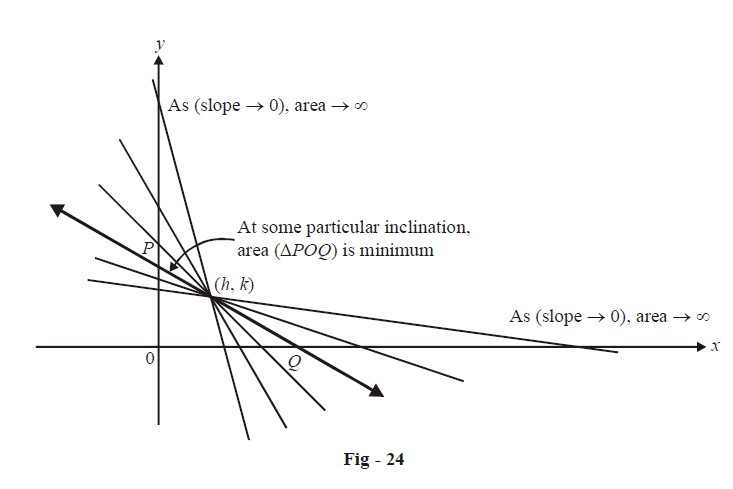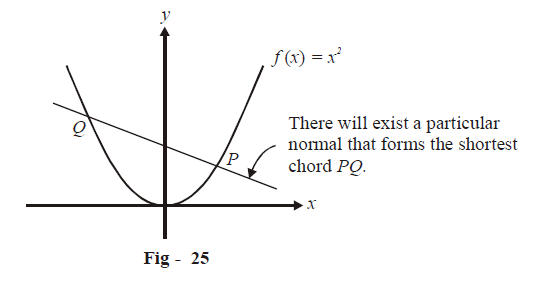# Examples on Maxima and Minima Set 2

Go back to  'Applications of Derivatives'

## Maxima and Minima Examples - Set 2

Example – 23

Let (h, k) be a fixed point, where h  > 0, k > 0. A straight line passing through this point cuts the positive direction of the co-ordinate axes at the points P and Q. Find the minimum area of $$\Delta OPQ,$$ O being the origin.

Solution: The given point (h, k) will lie in the first quadrant.

Convince yourself that there will be a particular slope of PQ at which the area of $$\Delta OPQ,$$ is minimum. If the $$\left( {{\rm{slope}}} \right) \to 0$$  or $$\left( {{\rm{slope}}} \right) \to \infty$$, $$\left( {{\rm{area}}} \right) \to \infty$$. However, at some finite slope in between these two extremes, area will assume a minimum value.Assume m to be the slope we wish to determine so that area is minimum (Notice that m will be negative). We first write down the equation of a straight line passing through (h, k) with slope m:

$y-k=m\left( x-h \right)$

This cuts the X-axis at \begin{align}P\left( {h - \frac{k}{m},0} \right)\end{align}  and the Y-axis at $$Q (0, k -mh)$$

Assume A to be the area of $$\Delta OPQ$$.

Therefore,

\begin{align}A &= \frac{1}{2} \times OP \times OQ\\ &= \frac{1}{2} \times \left( {h - \frac{k}{m}} \right) \times \left( {k - mh} \right)\\&= \frac{{ - 1}}{{2m}}{\left( {k - mh} \right)^2} \qquad \qquad \dots \rm{(i)}\end{align}

For A to be minimum,

\begin{align}\frac{{dA}}{{dm}} &= 0\,\,\,{\text{and}}\;\,\frac{{{d^2}A}}{{{d^2}m}} > 0\\\\\Rightarrow \qquad \frac{{dA}}{{dm}} &= \frac{{ - 1}}{{2m}}.2\left( {k - mh} \right).\left( { - h} \right) + {\left( {k - mh} \right)^2}.\frac{1}{{2{m^2}}}\\\\ &= \frac{1}{{2{m^2}}}\left( {{k^2} - {m^2}{h^2}} \right)\\\\\Rightarrow \qquad \frac{{dA}}{{dm}} &= 0\,\,\,{\text{when }}{k^2} - {m^2}{h^2} = 0\\\\\Rightarrow \qquad m &= \pm \frac{k}{h}\end{align}

Since m must be negative, \begin{align}m = \frac{{ - k}}{h}.\end{align}

Now,

\begin{align}& {{\left. \frac{{{d}^{2}}A}{d{{m}^{2}}} \right|}_{m=\frac{-k}{h}}}{{\left. =\frac{1}{2{{m}^{2}}}\left( -2m{{h}^{2}} \right)-\frac{\left( {{k}^{2}}-{{m}^{2}}{{h}^{2}} \right)}{{{m}^{3}}} \right|}_{m=\frac{-k}{h}}} \\ & \qquad\qquad\quad=\frac{{{h}^{3}}}{k}>0 \\ & \quad\Rightarrow\qquad \text{m}=\frac{-k}{h}\quad \text{ is a point of local minimum for A}\text{.} \\ \end{align}

From (i), the minimum value of A is:

\begin{align}{\left. {{A_{\min }} = \frac{{ - 1}}{{2m}}{{\left( {k - mh} \right)}^2}} \right|_{m = \frac{{ - k}}{h}}} = 2hk\end{align}

Example – 24

What normal to the curve $$y = {x^2}$$ forms the shortest chord?

Solution: As in the previous example, notice that there will exist a particular normal for which the  chord intercepted by the parabola is minimum. The maximum length of this chord is of course unbounded (infinity).Let us assume P to have the co-ordinates $$\left( {t,{t^2}} \right)$$. We will write the equation of the normal at P, find the other intersection point (the point Q) of this normal with the parabola, and then find PQ in terms of t. Then we will find t for which PQ is minimum.

\begin{align}&y = {x^2}\\\\\Rightarrow \qquad &{\left. {\frac{{dy}}{{dx}}} \right|_P} = {\left. {2x} \right|_P} = 2t\\\\ \Rightarrow \qquad &{m_N} = \frac{{ - 1}}{{2t}}\end{align}

{\rm\bf{Equation\;of\; normal:}}\qquad y - {t^2} =\begin{align} \frac{{ - 1}}{{2t}}\left( {x - t} \right) \qquad \qquad \dots\rm{(1)}\end{align}

Let the point Q be  $$\left( {t',t{'^2}} \right)$$. Since Q lies on the normal at P, the co-ordinates of Q must satisfy (i).

\begin{align} \Rightarrow \qquad &{t'^2} - {t^2} = \frac{{ - 1}}{{2t}}\left( {t' - t} \right)\\ \Rightarrow \qquad &\left( {t' + t} \right)\left( {t' - t} \right) = \frac{{ - 1}}{{2t}}\left( {t' - t} \right)\\ \Rightarrow \qquad &t' + t = \frac{{ - 1}}{{2t}}\left( {∵ t' - t \ne 0} \right)\\\Rightarrow \qquad &t' = - t - \frac{1}{{2t}}\end{align}

The length PQ is given by:

\begin{align}P{Q^2} &= {\left( {t' - t} \right)^2} + {\left( {{{t'}^2} - {t^2}} \right)^2}\\&= {\left( {t' - t} \right)^2}\left\{ {1 + {{\left( {t' + t} \right)}^2}} \right\}\\ &= {\left( {2t + \frac{1}{{2t}}} \right)^2}\left\{ {1 + \frac{1}{{4{t^2}}}} \right\}\\&= 4{t^2}{\left( {1 + \frac{1}{{4{t^2}}}} \right)^3}\end{align}

To minimize PQ, we can equivalently minimize $$P{Q^2}$$.

\begin{align} \Rightarrow \frac{{d{{\left( {PQ} \right)}^2}}}{{dt}}& = 8t{\left( {1 + \frac{1}{{4{t^2}}}} \right)^3} + 4{t^2} \times 3{\left( {1 + \frac{1}{{4{t^2}}}} \right)^2} \times \frac{{ - 2}}{{4{t^3}}}\\\\ &= 4\left( {1 + \frac{1}{{4{t^2}}}} \right)\left( {\frac{{2{t^2} - 1}}{t}} \right)\end{align}

This is 0 when \begin{align}{t^2} = \frac{1}{2}{\rm{\;\; or\;\; }}t = \pm \frac{1}{{\sqrt 2 }} \end{align}

\begin{align}\text{Verify that} {\left. { \frac{{{d^2}\left( {P{Q^2}} \right)}}{{d{t^2}}}} \right|_{t = \pm \frac{1}{{\sqrt 2 }}}} > 0\\ \Rightarrow \qquad PQ\; \text{is minimum for t} = \pm \frac{1}{{\sqrt 2 }}\end{align}

Finding out the equations of the normals corresponding to the two values of t is left to the reader as an exercise.